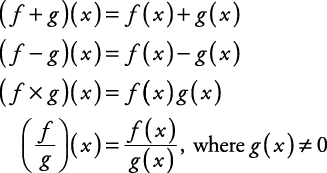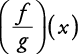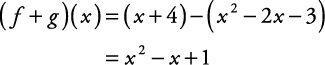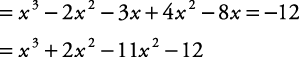## Algebra of Functions

If two functions have a common domain, then arithmetic can be performed with them using the following definitions.##### Example 1

If f ( x) = x + 4 and g( x) = x 2 – 2 x – 3, find each of the following and determine the common domain.

1. ( f + g)( x)

2. ( fg)( x)

3. ( f × g)( x)

4.5.The common domain is {all real numbers}.

1.The common domain is {all real numbers}.

1. ( f × g)( x) = ( x + 4) × ( x 2 – 2 x – 3)

Use the distributive property.The common domain is {all real numbers}.

1.This expression is undefined when x = 3 or when x = –1. So the common domain is {all real numbers except 3 or –1}.Back to Top
A18ACD436D5A3997E3DA2573E3FD792A